# 算法介绍

A*（念做：A Star）算法是一种很常用的路径查找和图形遍历算法。它有较好的性能和准确度。本文在讲解算法的同时也会提供Python语言的代码实现，并会借助matplotlib库动态的展示算法的运算过程。

A*算法最初发表于1968年，由Stanford研究院的Peter Hart, Nils Nilsson以及Bertram Raphael发表。它可以被认为是Dijkstra算法的扩展。

# 广度优先搜索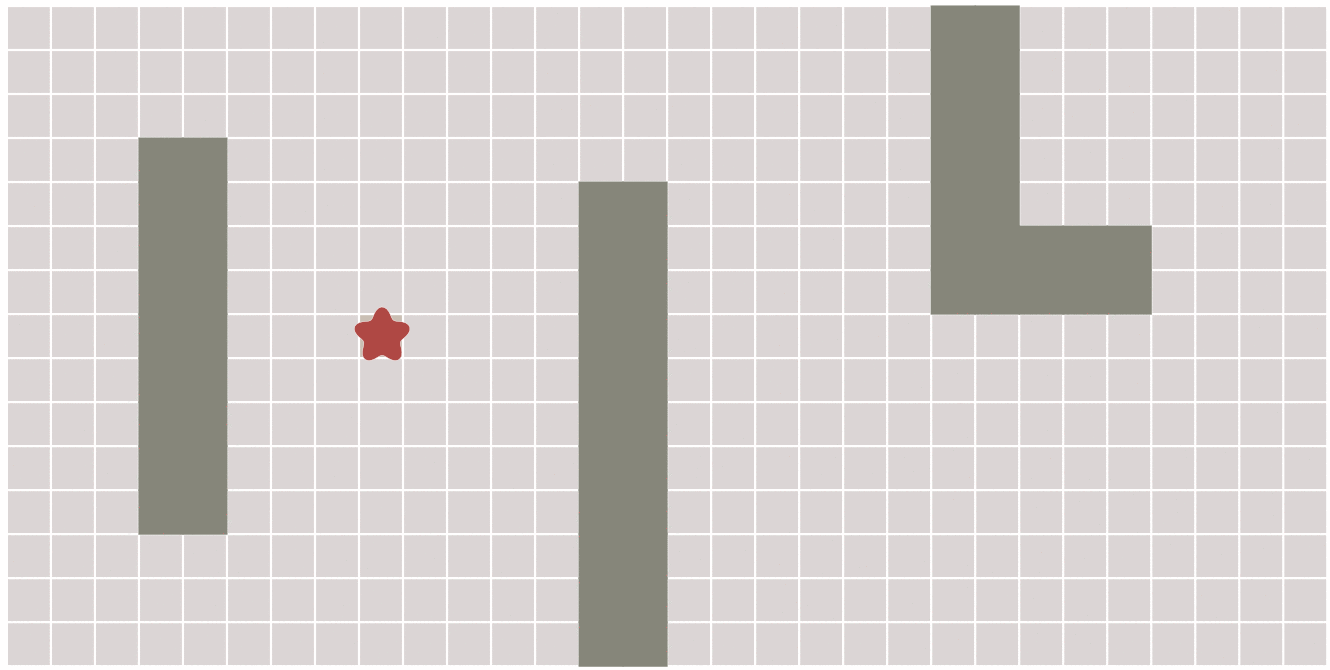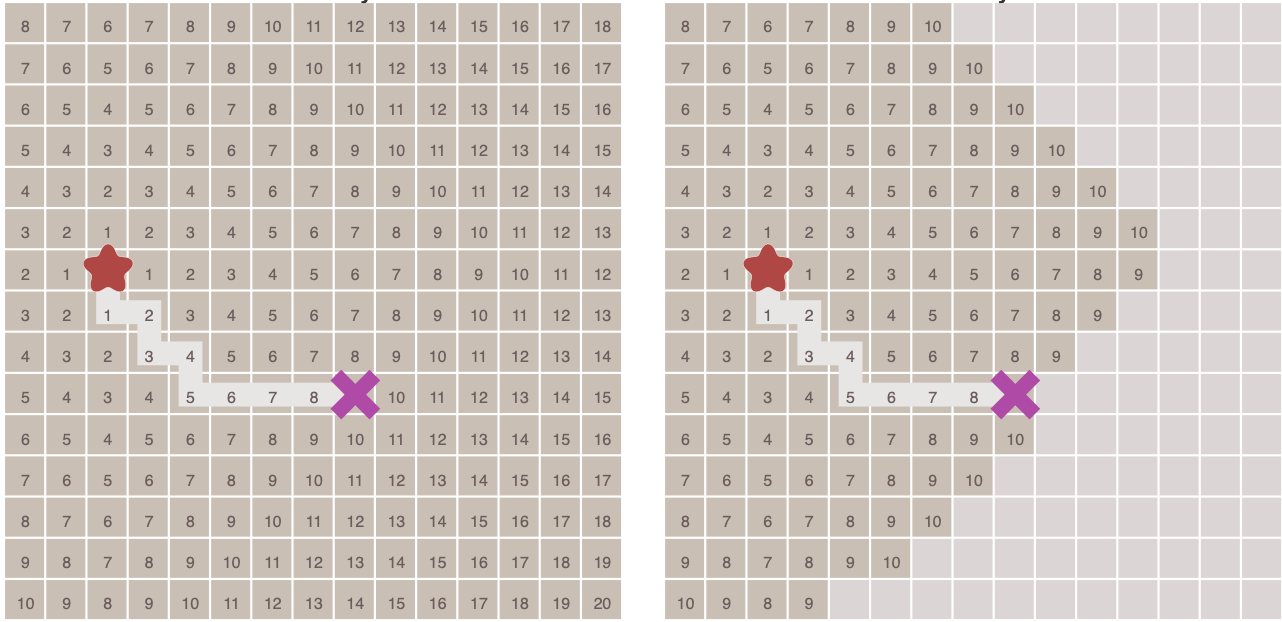# Dijkstra算法

Dijkstra算法是由计算机科学家Edsger W. Dijkstra在1956年提出的。

Dijkstra算法用来寻找图形中节点之间的最短路径。# 最佳优先搜索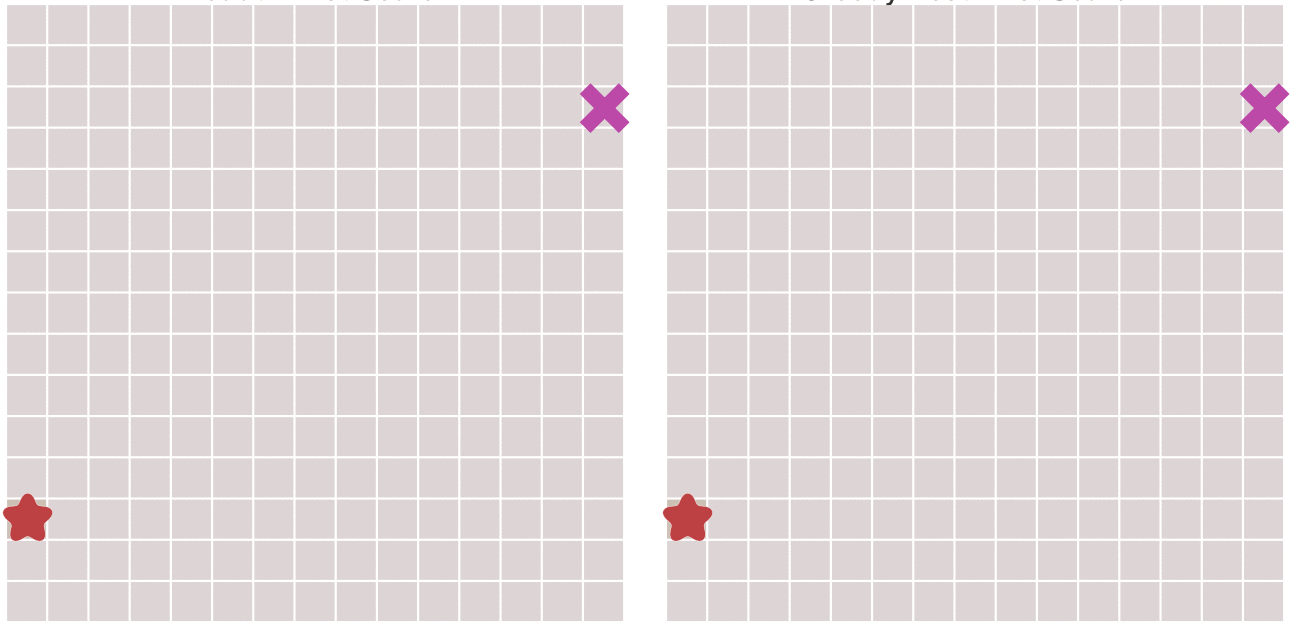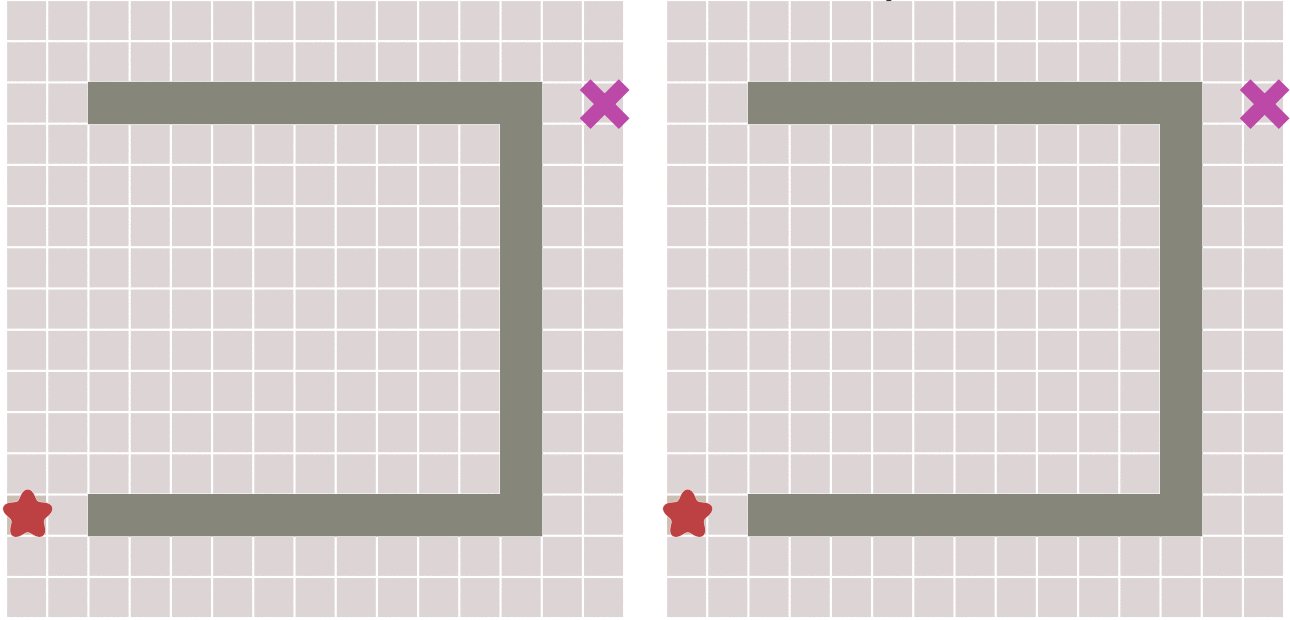# A*算法

A*算法通过下面这个函数来计算每个节点的优先级。

$$f(n) = g(n) + h(n)$$

• $f(n)$ 是节点n的综合优先级。当我们选择下一个要遍历的节点时，我们总会选取综合优先级最高（值最小）的节点。
• $g(n)$ 是节点n距离起点的代价。
• $h(n)$ 是节点n距离终点的预计代价，这也就是A*算法的启发函数。关于启发函数我们在下面详细讲解。

A*算法在运算过程中，每次从优先队列中选取$f(n)$值最小（优先级最高）的节点作为下一个待遍历的节点。

* 初始化open_set和close_set；
* 将起点加入open_set中，并设置优先级为0（优先级最高）；
* 如果open_set不为空，则从open_set中选取优先级最高的节点n：
* 如果节点n为终点，则：
* 从终点开始逐步追踪parent节点，一直达到起点；
* 返回找到的结果路径，算法结束；
* 如果节点n不是终点，则：
* 将节点n从open_set中删除，并加入close_set中；
* 遍历节点n所有的邻近节点：
* 如果邻近节点m在close_set中，则：
* 跳过，选取下一个邻近节点
* 如果邻近节点m也不在open_set中，则：
* 设置节点m的parent为节点n
* 计算节点m的优先级
* 将节点m加入open_set中

## 启发函数

• 在极端情况下，当启发函数$h(n)$始终为0，则将由$g(n)$决定节点的优先级，此时算法就退化成了Dijkstra算法。
• 如果$h(n)$始终小于等于节点n到终点的代价，则A*算法保证一定能够找到最短路径。但是当$h(n)$的值越小，算法将遍历越多的节点，也就导致算法越慢。
• 如果$h(n)$完全等于节点n到终点的代价，则A*算法将找到最佳路径，并且速度很快。可惜的是，并非所有场景下都能做到这一点。因为在没有达到终点之前，我们很难确切算出距离终点还有多远。
• 如果$h(n)$的值比节点n到终点的代价要大，则A*算法不能保证找到最短路径，不过此时会很快。
• 在另外一个极端情况下，如果$h(n)$相较于$g(n)$大很多，则此时只有$h(n)$产生效果，这也就变成了最佳优先搜索。

• 如果图形中只允许朝上下左右四个方向移动，则可以使用曼哈顿距离（Manhattan distance）。
• 如果图形中允许朝八个方向移动，则可以使用对角距离。
• 如果图形中允许朝任何方向移动，则可以使用欧几里得距离（Euclidean distance）。

## 关于距离

### 曼哈顿距离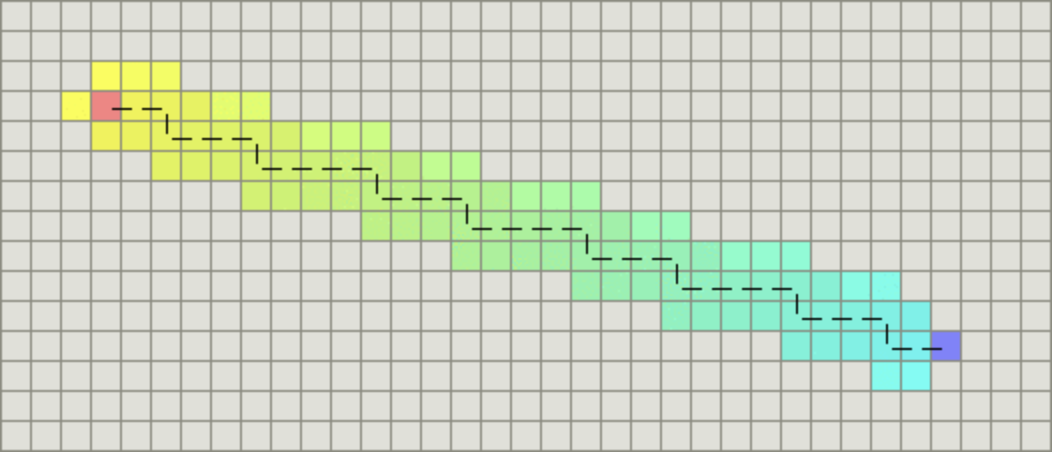function heuristic(node) =
dx = abs(node.x - goal.x)
dy = abs(node.y - goal.y)
return D * (dx + dy)

### 对角距离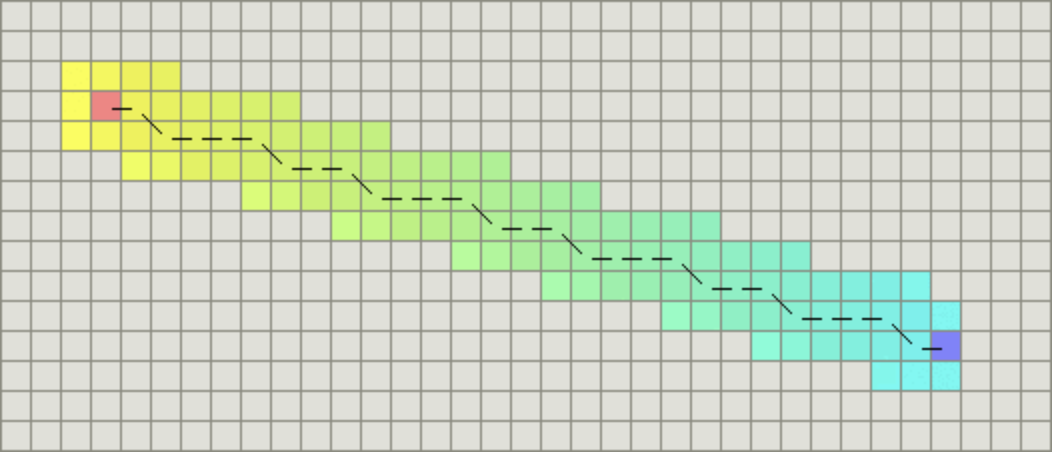function heuristic(node) =
dx = abs(node.x - goal.x)
dy = abs(node.y - goal.y)
return D * (dx + dy) + (D2 - 2 * D) * min(dx, dy)

### 欧几里得距离

function heuristic(node) =
dx = abs(node.x - goal.x)
dy = abs(node.y - goal.y)
return D * sqrt(dx * dx + dy * dy)

# 算法实现

## 坐标点与地图

# point.py

import sys

class Point:
def __init__(self, x, y):
self.x = x
self.y = y
self.cost = sys.maxsize

# random_map.py

import numpy as np

import point

class RandomMap:
def __init__(self, size=50): ①
self.size = size
self.obstacle = size//8 ②
self.GenerateObstacle() ③

def GenerateObstacle(self):
self.obstacle_point = []
self.obstacle_point.append(point.Point(self.size//2, self.size//2))
self.obstacle_point.append(point.Point(self.size//2, self.size//2-1))

# Generate an obstacle in the middle
for i in range(self.size//2-4, self.size//2): ④
self.obstacle_point.append(point.Point(i, self.size-i))
self.obstacle_point.append(point.Point(i, self.size-i-1))
self.obstacle_point.append(point.Point(self.size-i, i))
self.obstacle_point.append(point.Point(self.size-i, i-1))

for i in range(self.obstacle-1): ⑤
x = np.random.randint(0, self.size)
y = np.random.randint(0, self.size)
self.obstacle_point.append(point.Point(x, y))

if (np.random.rand() > 0.5): # Random boolean ⑥
for l in range(self.size//4):
self.obstacle_point.append(point.Point(x, y+l))
pass
else:
for l in range(self.size//4):
self.obstacle_point.append(point.Point(x+l, y))
pass

def IsObstacle(self, i ,j): ⑦
for p in self.obstacle_point:
if i==p.x and j==p.y:
return True
return False

1. 构造函数，地图的默认大小是50x50；
2. 设置障碍物的数量为地图大小除以8；
3. 调用GenerateObstacle生成随机障碍物；
4. 在地图的中间生成一个斜着的障碍物；
5. 随机生成其他几个障碍物；
6. 障碍物的方向也是随机的；
7. 定义一个方法来判断某个节点是否是障碍物；

## 算法主体

# a_star.py

import sys
import time

import numpy as np

from matplotlib.patches import Rectangle

import point
import random_map

class AStar:
def __init__(self, map):
self.map=map
self.open_set = []
self.close_set = []

def BaseCost(self, p):
x_dis = p.x
y_dis = p.y
# Distance to start point
return x_dis + y_dis + (np.sqrt(2) - 2) * min(x_dis, y_dis)

def HeuristicCost(self, p):
x_dis = self.map.size - 1 - p.x
y_dis = self.map.size - 1 - p.y
# Distance to end point
return x_dis + y_dis + (np.sqrt(2) - 2) * min(x_dis, y_dis)

def TotalCost(self, p):
return self.BaseCost(p) + self.HeuristicCost(p)

def IsValidPoint(self, x, y):
if x < 0 or y < 0:
return False
if x >= self.map.size or y >= self.map.size:
return False
return not self.map.IsObstacle(x, y)

def IsInPointList(self, p, point_list):
for point in point_list:
if point.x == p.x and point.y == p.y:
return True
return False

def IsInOpenList(self, p):
return self.IsInPointList(p, self.open_set)

def IsInCloseList(self, p):
return self.IsInPointList(p, self.close_set)

def IsStartPoint(self, p):
return p.x == 0 and p.y ==0

def IsEndPoint(self, p):
return p.x == self.map.size-1 and p.y == self.map.size-1

• __init__：类的构造函数。
• BaseCost：节点到起点的移动代价，对应了上文的$g(n)$。
• HeuristicCost：节点到终点的启发函数，对应上文的$h(n)$。由于我们是基于网格的图形，所以这个函数和上一个函数用的是对角距离。
• TotalCost：代价总和，即对应上面提到的$f(n)$。
• IsValidPoint：判断点是否有效，不在地图内部或者障碍物所在点都是无效的。
• IsInPointList：判断点是否在某个集合中。
• IsInOpenList：判断点是否在open_set中。
• IsInCloseList：判断点是否在close_set中。
• IsStartPoint：判断点是否是起点。
• IsEndPoint：判断点是否是终点。

# a_star.py
def RunAndSaveImage(self, ax, plt):
start_time = time.time()

start_point = point.Point(0, 0)
start_point.cost = 0
self.open_set.append(start_point)

while True:
index = self.SelectPointInOpenList()
if index < 0:
print('No path found, algorithm failed!!!')
return
p = self.open_set[index]
rec = Rectangle((p.x, p.y), 1, 1, color='c')
ax.add_patch(rec)
self.SaveImage(plt)

if self.IsEndPoint(p):
return self.BuildPath(p, ax, plt, start_time)

del self.open_set[index]
self.close_set.append(p)

# Process all neighbors
x = p.x
y = p.y
self.ProcessPoint(x-1, y+1, p)
self.ProcessPoint(x-1, y, p)
self.ProcessPoint(x-1, y-1, p)
self.ProcessPoint(x, y-1, p)
self.ProcessPoint(x+1, y-1, p)
self.ProcessPoint(x+1, y, p)
self.ProcessPoint(x+1, y+1, p)
self.ProcessPoint(x, y+1, p)

# a_star.py
def SaveImage(self, plt):
millis = int(round(time.time() * 1000))
filename = './' + str(millis) + '.png'
plt.savefig(filename)

def ProcessPoint(self, x, y, parent):
if not self.IsValidPoint(x, y):
return # Do nothing for invalid point
p = point.Point(x, y)
if self.IsInCloseList(p):
return # Do nothing for visited point
print('Process Point [', p.x, ',', p.y, ']', ', cost: ', p.cost)
if not self.IsInOpenList(p):
p.parent = parent
p.cost = self.TotalCost(p)
self.open_set.append(p)

def SelectPointInOpenList(self):
index = 0
selected_index = -1
min_cost = sys.maxsize
for p in self.open_set:
cost = self.TotalCost(p)
if cost < min_cost:
min_cost = cost
selected_index = index
index += 1
return selected_index

def BuildPath(self, p, ax, plt, start_time):
path = []
while True:
path.insert(0, p) # Insert first
if self.IsStartPoint(p):
break
else:
p = p.parent
for p in path:
rec = Rectangle((p.x, p.y), 1, 1, color='g')
ax.add_patch(rec)
plt.draw()
self.SaveImage(plt)
end_time = time.time()
print('===== Algorithm finish in', int(end_time-start_time), ' seconds')

• SaveImage：将当前状态保存到图片中，图片以当前时间命名。
• ProcessPoint：针对每一个节点进行处理：如果是没有处理过的节点，则计算优先级设置父节点，并且添加到open_set中。
• SelectPointInOpenList：从open_set中找到优先级最高的节点，返回其索引。
• BuildPath：从终点往回沿着parent构造结果路径。然后从起点开始绘制结果，结果使用绿色方块，每次绘制一步便保存一个图片。

## 测试入口

# main.py

import numpy as np
import matplotlib.pyplot as plt

from matplotlib.patches import Rectangle

import random_map
import a_star

plt.figure(figsize=(5, 5))

map = random_map.RandomMap() ①

ax = plt.gca()
ax.set_xlim([0, map.size]) ②
ax.set_ylim([0, map.size])

for i in range(map.size): ③
for j in range(map.size):
if map.IsObstacle(i,j):
rec = Rectangle((i, j), width=1, height=1, color='gray')
ax.add_patch(rec)
else:
rec = Rectangle((i, j), width=1, height=1, edgecolor='gray', facecolor='w')
ax.add_patch(rec)

rec = Rectangle((0, 0), width = 1, height = 1, facecolor='b')
ax.add_patch(rec) ④

rec = Rectangle((map.size-1, map.size-1), width = 1, height = 1, facecolor='r')
ax.add_patch(rec) ⑤

plt.axis('equal') ⑥
plt.axis('off')
plt.tight_layout()
#plt.show()

a_star = a_star.AStar(map)
a_star.RunAndSaveImage(ax, plt) ⑦

1. 创建一个随机地图；
2. 设置图像的内容与地图大小一致；
3. 绘制地图：对于障碍物绘制一个灰色的方块，其他区域绘制一个白色的的方块；
4. 绘制起点为蓝色方块；
5. 绘制终点为红色方块；
6. 设置图像的坐标轴比例相等并且隐藏坐标轴；
7. 调用算法来查找路径；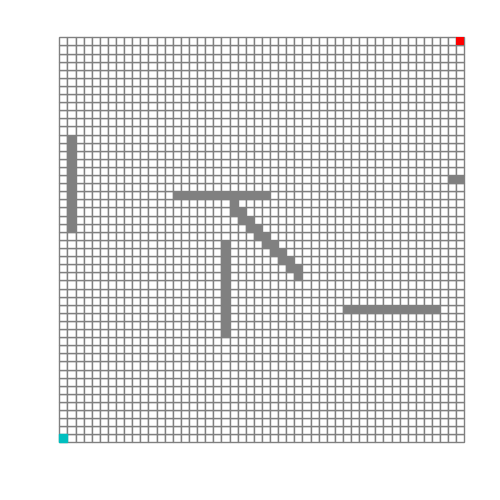# 算法变种

A*算法有不少的变种，这里我们介绍最主要的几个。

## ARA*

ARA 全称是Anytime Repairing A*，也称为Anytime A

## D*

D*是Dynamic A*的简写，其算法和A*类似，不同的是，其代价的计算在算法运行过程中可能会发生变化。

D*包含了下面三种增量搜索算法：

• 原始的D*由Anthony Stentz发表。
• Focussed D*由Anthony Stentz发表，是一个增量启发式搜索算法，结合了A*和原始D*的思想。
• D Lite是由Sven Koenig和Maxim Likhachev基于LPA构建的算法。

D*及其变体已广泛用于移动机器人和自动车辆导航。当前系统通常基于D* Lite而不是原始D*或Focussed D*。

## Field D*

Field D*扩展了D*和D* Lite，是一种基于插值（ interpolation-based ）的规划算法，它使用线性插值来有效地生成低成本路径，从而消除不必要的转向。

## Block A*

Block A*扩展自A*，但它操作是一块（block）单元而不是单个单元。

open_set中块的优先级称为其堆值（heap value）。与A*类似，Block A*中的基本循环是删除具有最低堆值的条目并将其展开。在扩展期间使用LDDB来计算正在扩展的块中的边界单元的g值。

LDDB是一种新型数据库，它包含了本地邻域边界点之间的距离。

# 参考资料与推荐读物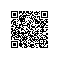使用钉钉扫一扫加入圈子
+ 订阅